# Plots and Trend Lines 4 5 Scatter Plots

• Slides: 32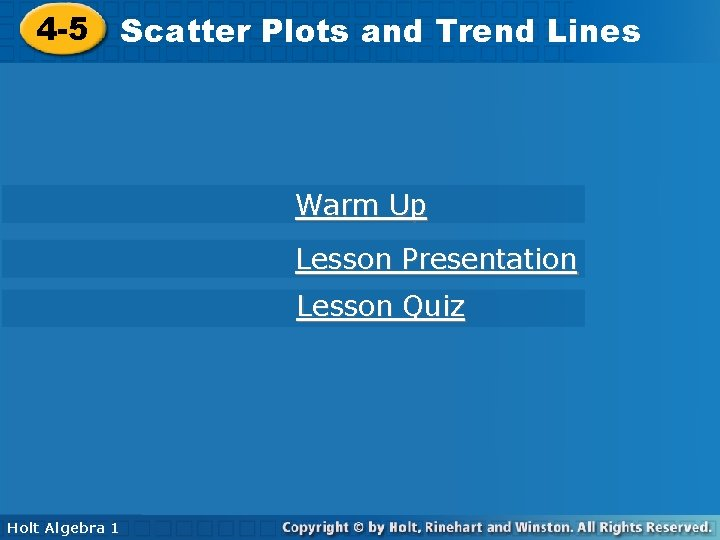Plots and Trend Lines 4 -5 Scatter Plots and Trend Lines Warm Up Lesson Presentation Lesson Quiz Holt Algebra 1 1 Holt Algebra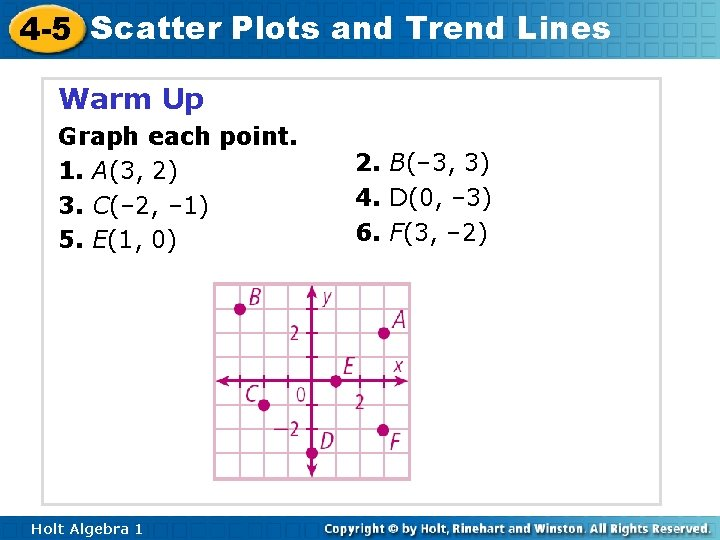4 -5 Scatter Plots and Trend Lines Warm Up Graph each point. 1. A(3, 2) 3. C(– 2, – 1) 5. E(1, 0) Holt Algebra 1 2. B(– 3, 3) 4. D(0, – 3) 6. F(3, – 2)4 -5 Scatter Plots and Trend Lines Objectives Create and interpret scatter plots. Use trend lines to make predictions. Holt Algebra 14 -5 Scatter Plots and Trend Lines Vocabulary scatter plot correlation positive correlation negative correlation no correlation trend line Holt Algebra 1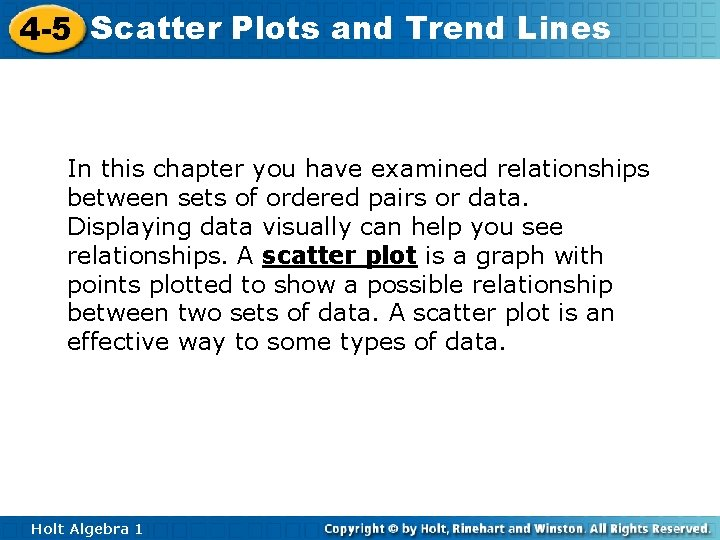4 -5 Scatter Plots and Trend Lines In this chapter you have examined relationships between sets of ordered pairs or data. Displaying data visually can help you see relationships. A scatter plot is a graph with points plotted to show a possible relationship between two sets of data. A scatter plot is an effective way to some types of data. Holt Algebra 1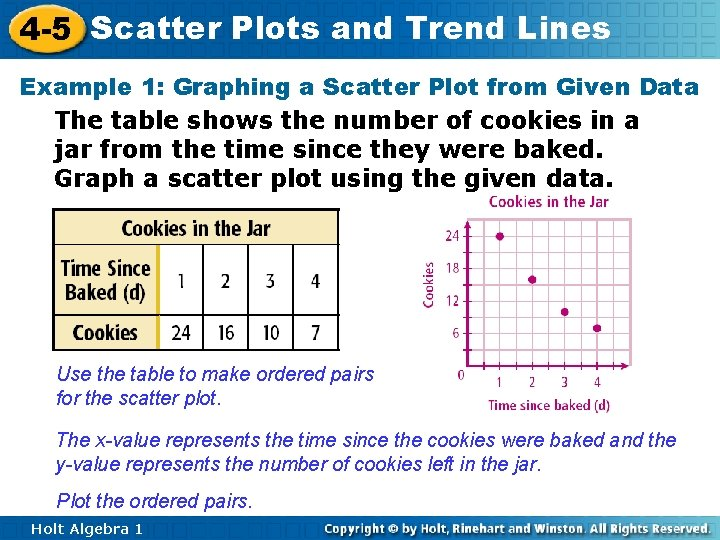4 -5 Scatter Plots and Trend Lines Example 1: Graphing a Scatter Plot from Given Data The table shows the number of cookies in a jar from the time since they were baked. Graph a scatter plot using the given data. Use the table to make ordered pairs for the scatter plot. The x-value represents the time since the cookies were baked and the y-value represents the number of cookies left in the jar. Plot the ordered pairs. Holt Algebra 1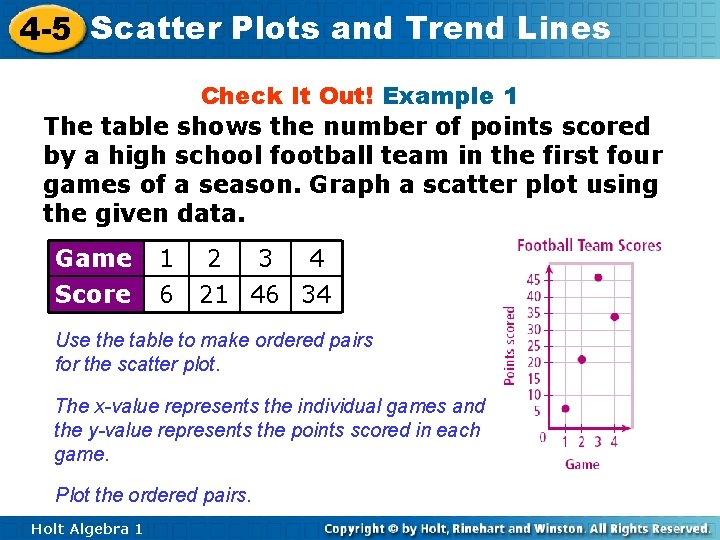4 -5 Scatter Plots and Trend Lines Check It Out! Example 1 The table shows the number of points scored by a high school football team in the first four games of a season. Graph a scatter plot using the given data. Game Score 1 6 2 3 4 21 46 34 Use the table to make ordered pairs for the scatter plot. The x-value represents the individual games and the y-value represents the points scored in each game. Plot the ordered pairs. Holt Algebra 14 -5 Scatter Plots and Trend Lines A correlation describes a relationship between two data sets. A graph may show the correlation between data. The correlation can help you analyze trends and make predictions. There are three types of correlations between data. Holt Algebra 1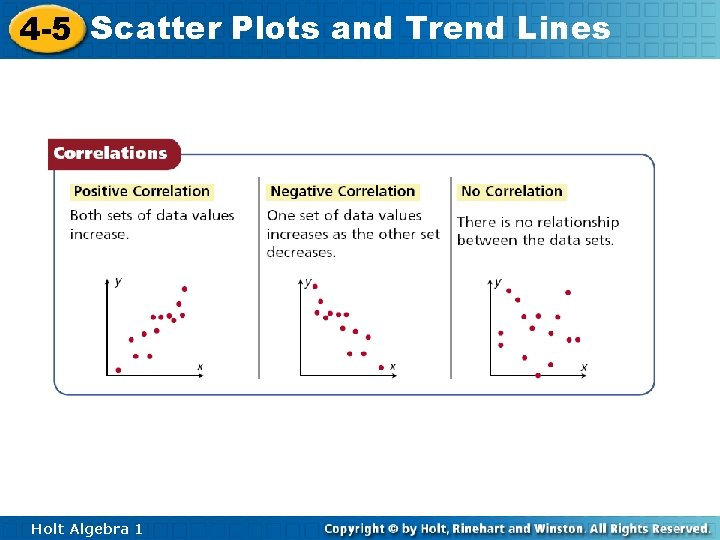4 -5 Scatter Plots and Trend Lines Holt Algebra 1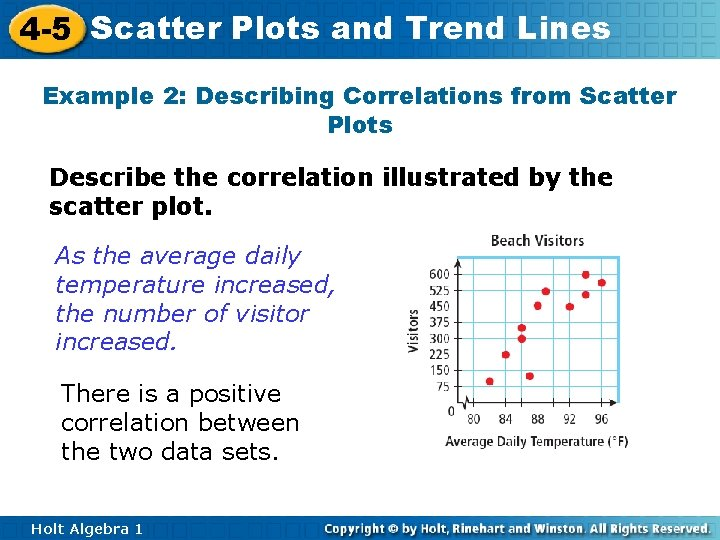4 -5 Scatter Plots and Trend Lines Example 2: Describing Correlations from Scatter Plots Describe the correlation illustrated by the scatter plot. As the average daily temperature increased, the number of visitor increased. There is a positive correlation between the two data sets. Holt Algebra 1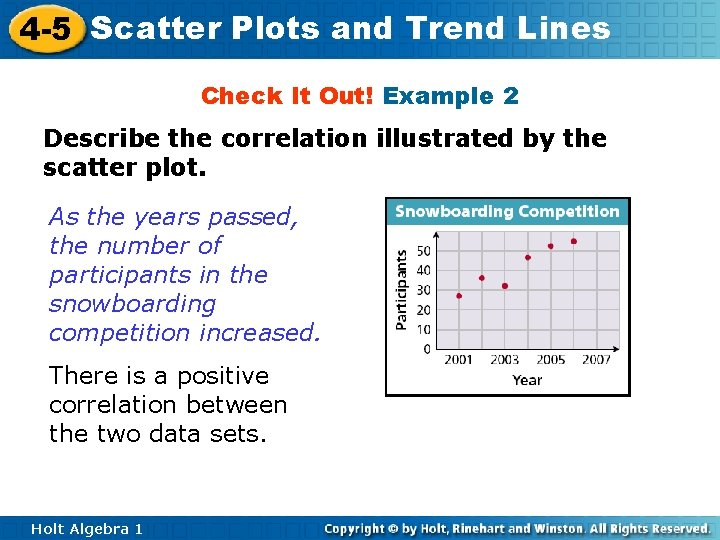4 -5 Scatter Plots and Trend Lines Check It Out! Example 2 Describe the correlation illustrated by the scatter plot. As the years passed, the number of participants in the snowboarding competition increased. There is a positive correlation between the two data sets. Holt Algebra 1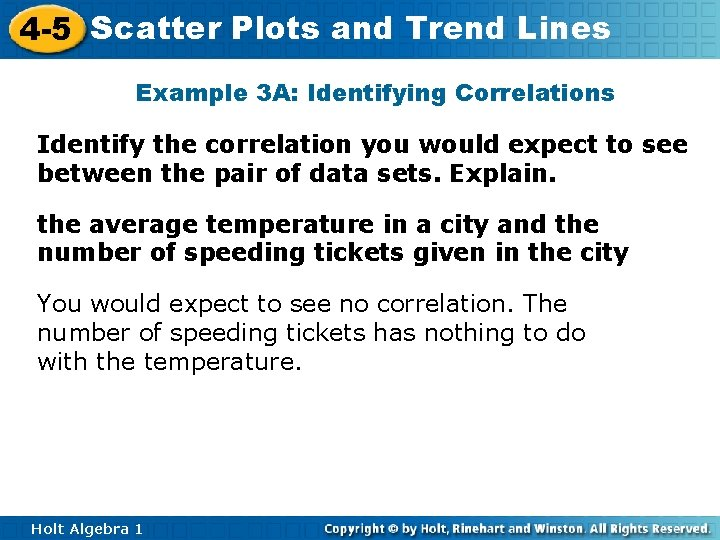4 -5 Scatter Plots and Trend Lines Example 3 A: Identifying Correlations Identify the correlation you would expect to see between the pair of data sets. Explain. the average temperature in a city and the number of speeding tickets given in the city You would expect to see no correlation. The number of speeding tickets has nothing to do with the temperature. Holt Algebra 1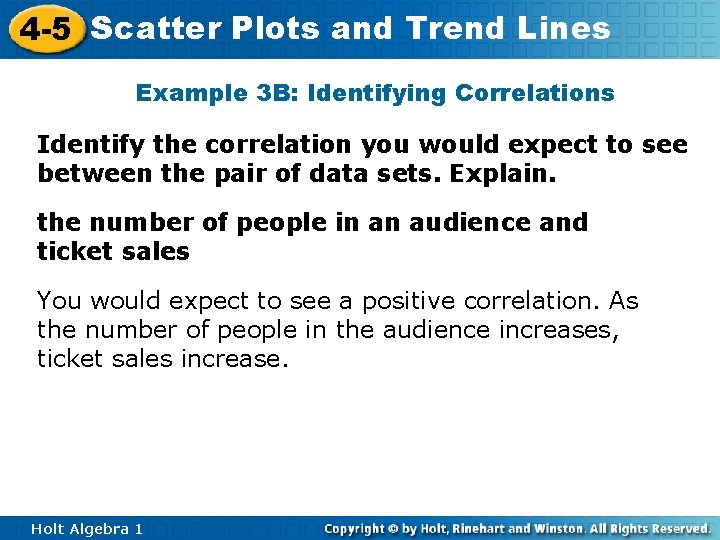4 -5 Scatter Plots and Trend Lines Example 3 B: Identifying Correlations Identify the correlation you would expect to see between the pair of data sets. Explain. the number of people in an audience and ticket sales You would expect to see a positive correlation. As the number of people in the audience increases, ticket sales increase. Holt Algebra 14 -5 Scatter Plots and Trend Lines Example 3 C: Identifying Correlations Identify the correlation you would expect to see between the pair of data sets. Explain. a runner’s time and the distance to the finish line You would expect to see a negative correlation. As a runner’s time increases, the distance to the finish line decreases. Holt Algebra 1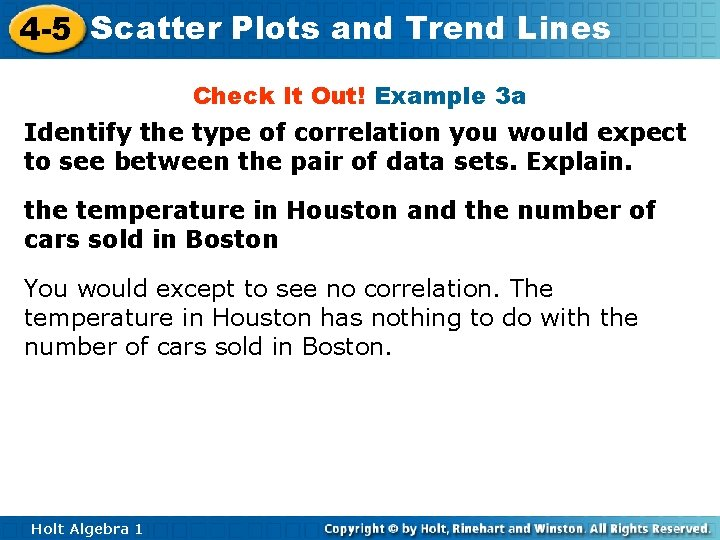4 -5 Scatter Plots and Trend Lines Check It Out! Example 3 a Identify the type of correlation you would expect to see between the pair of data sets. Explain. the temperature in Houston and the number of cars sold in Boston You would except to see no correlation. The temperature in Houston has nothing to do with the number of cars sold in Boston. Holt Algebra 1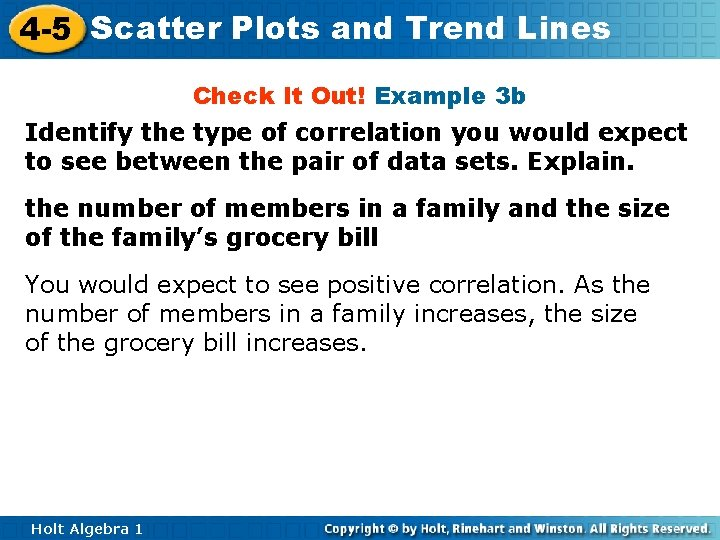4 -5 Scatter Plots and Trend Lines Check It Out! Example 3 b Identify the type of correlation you would expect to see between the pair of data sets. Explain. the number of members in a family and the size of the family’s grocery bill You would expect to see positive correlation. As the number of members in a family increases, the size of the grocery bill increases. Holt Algebra 1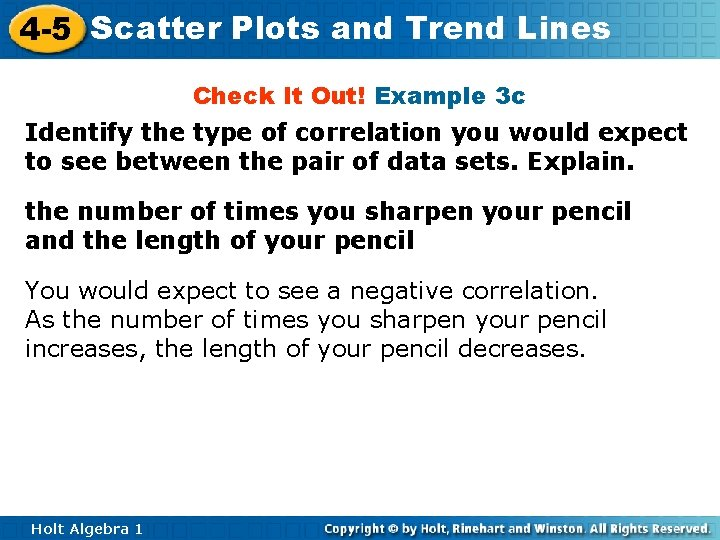4 -5 Scatter Plots and Trend Lines Check It Out! Example 3 c Identify the type of correlation you would expect to see between the pair of data sets. Explain. the number of times you sharpen your pencil and the length of your pencil You would expect to see a negative correlation. As the number of times you sharpen your pencil increases, the length of your pencil decreases. Holt Algebra 14 -5 Scatter Plots and Trend Lines Example 4: Matching Scatter Plots to Situations Choose the scatter plot that best represents the relationship between the age of a car and the amount of money spent each year on repairs. Explain. Graph A Holt Algebra 1 Graph B Graph C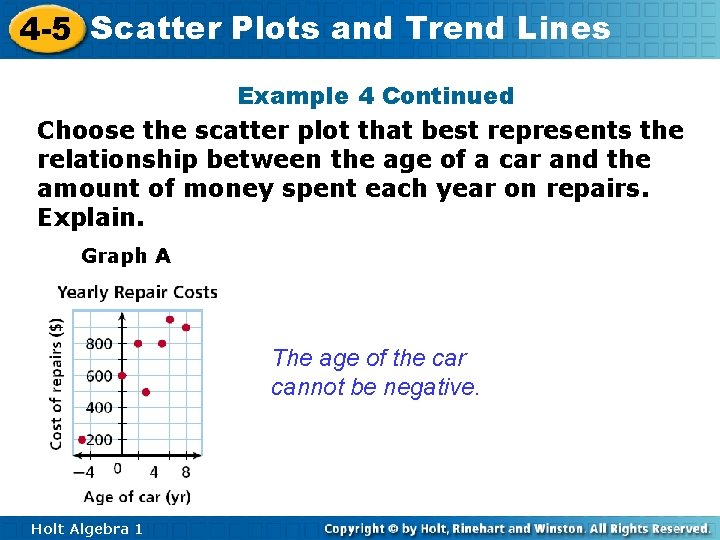4 -5 Scatter Plots and Trend Lines Example 4 Continued Choose the scatter plot that best represents the relationship between the age of a car and the amount of money spent each year on repairs. Explain. Graph A The age of the car cannot be negative. Holt Algebra 14 -5 Scatter Plots and Trend Lines Example 4 Continued Choose the scatter plot that best represents the relationship between the age of a car and the amount of money spent each year on repairs. Explain. Graph B This graph shows all positive values and a positive correlation, so it could represent the data set. Holt Algebra 1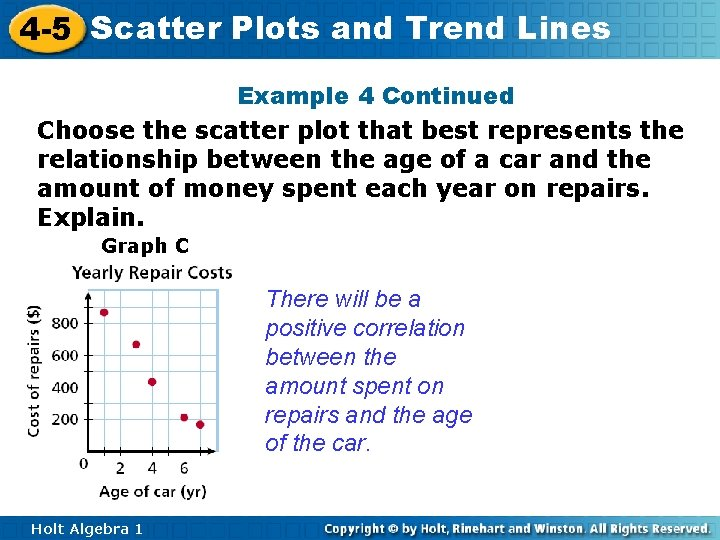4 -5 Scatter Plots and Trend Lines Example 4 Continued Choose the scatter plot that best represents the relationship between the age of a car and the amount of money spent each year on repairs. Explain. Graph C There will be a positive correlation between the amount spent on repairs and the age of the car. Holt Algebra 1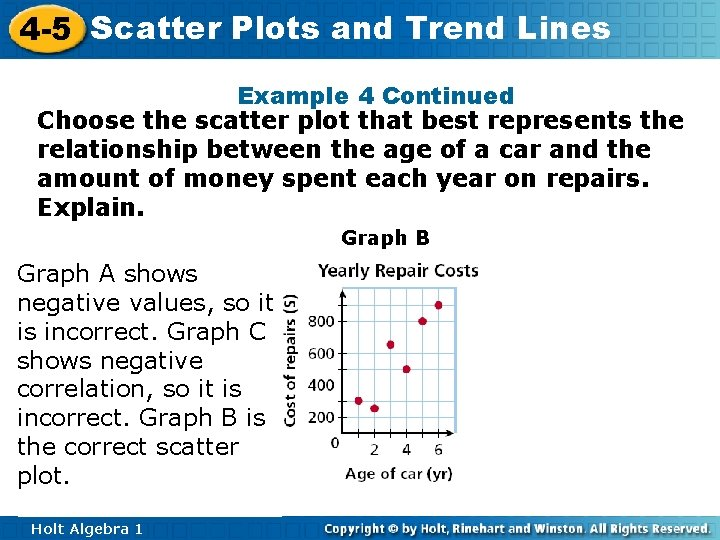4 -5 Scatter Plots and Trend Lines Example 4 Continued Choose the scatter plot that best represents the relationship between the age of a car and the amount of money spent each year on repairs. Explain. Graph A shows negative values, so it is incorrect. Graph C shows negative correlation, so it is incorrect. Graph B is the correct scatter plot. Holt Algebra 1 Graph B Graph C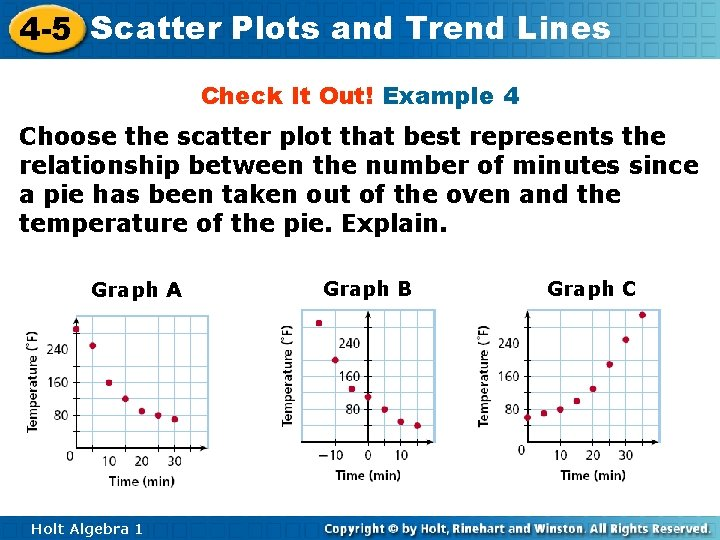4 -5 Scatter Plots and Trend Lines Check It Out! Example 4 Choose the scatter plot that best represents the relationship between the number of minutes since a pie has been taken out of the oven and the temperature of the pie. Explain. Graph A Holt Algebra 1 Graph B Graph C4 -5 Scatter Plots and Trend Lines Check It Out! Example 4 Continued Choose the scatter plot that best represents the relationship between the number of minutes since a pie has been taken out of the oven and the temperature of the pie. Explain. Graph A The pie is cooling steadily after it is take from the oven. Holt Algebra 14 -5 Scatter Plots and Trend Lines Check It Out! Example 4 Continued Choose the scatter plot that best represents the relationship between the number of minutes since a pie has been taken out of the oven and the temperature of the pie. Explain. Graph B The pie has started cooling before it is taken from the oven. Holt Algebra 14 -5 Scatter Plots and Trend Lines Check It Out! Example 4 Continued Choose the scatter plot that best represents the relationship between the number of minutes since a pie has been taken out of the oven and the temperature of the pie. Explain. Graph C The temperature of the pie is increasing after it is taken from the oven. Holt Algebra 1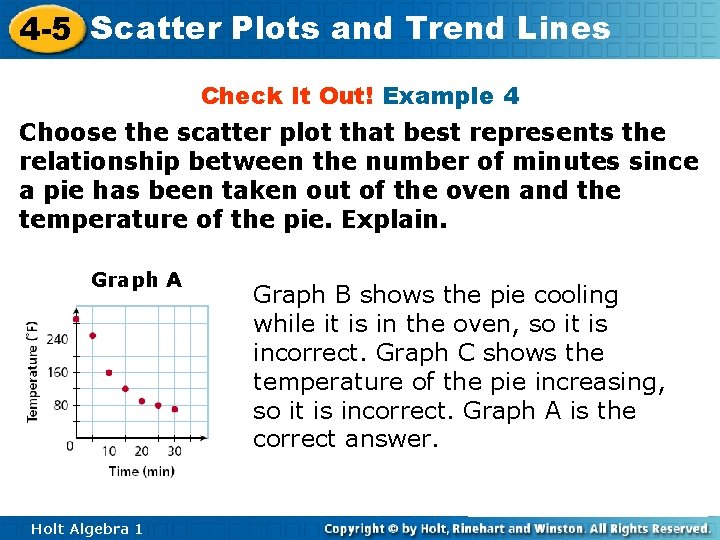4 -5 Scatter Plots and Trend Lines Check It Out! Example 4 Choose the scatter plot that best represents the relationship between the number of minutes since a pie has been taken out of the oven and the temperature of the pie. Explain. Graph A Holt Algebra 1 Graph B Graph C Graph B shows the pie cooling while it is in the oven, so it is incorrect. Graph C shows the temperature of the pie increasing, so it is incorrect. Graph A is the correct answer.4 -5 Scatter Plots and Trend Lines You can graph a function on a scatter plot to help show a relationship in the data. Sometimes the function is a straight line. This line, called a trend line, helps show the correlation between data sets more clearly. It can also be helpful when making predictions based on the data. Holt Algebra 14 -5 Scatter Plots and Trend Lines Example 5: Fund-Raising Application The scatter plot shows a relationship between the total amount of money collected at the concession stand the total number of tickets sold at a movie theater. Based on this relationship, predict how much money will be collected at the concession stand when 150 tickets have been sold. Draw a trend line and use it to make a prediction. Draw a line that has about the same number of points above and below it. Your line may or may not go through data points. Find the point on the line whose x-value is 150. The corresponding y-value is 750. Based on the data, \$750 is a reasonable prediction of how much money will be collected when 150 tickets have been sold. Holt Algebra 1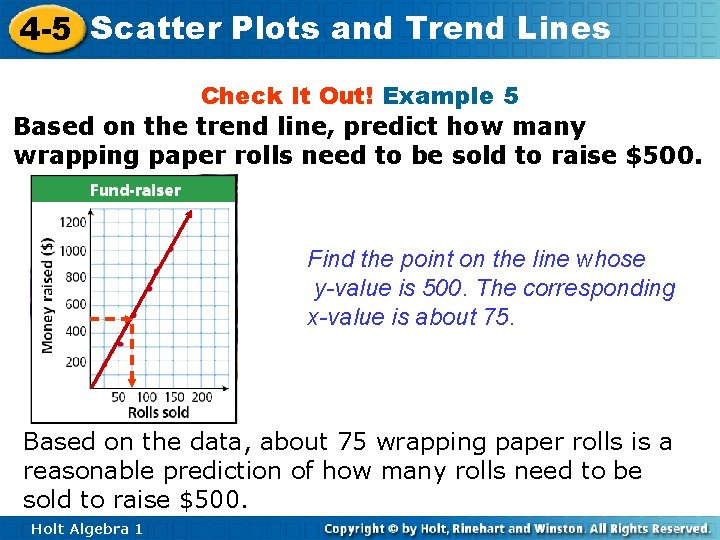4 -5 Scatter Plots and Trend Lines Check It Out! Example 5 Based on the trend line, predict how many wrapping paper rolls need to be sold to raise \$500. Find the point on the line whose y-value is 500. The corresponding x-value is about 75. Based on the data, about 75 wrapping paper rolls is a reasonable prediction of how many rolls need to be sold to raise \$500. Holt Algebra 14 -5 Scatter Plots and Trend Lines Lesson Quiz: Part I For Items 1 and 2, identify the correlation you would expect to see between each pair of data sets. Explain. 1. The outside temperature in the summer and the cost of the electric bill Positive correlation; as the outside temperature increases, the electric bill increases because of the air conditioner. 2. The price of a car and the number of passengers it seats No correlation; a very expensive car could seat only 2 passengers. Holt Algebra 14 -5 Scatter Plots and Trend Lines Lesson Quiz: Part II 3. The scatter plot shows the number of orders placed for flowers before Valentine’s Day at one shop. Based on this relationship, predict the number of flower orders placed on February 12. about 45 Holt Algebra 1